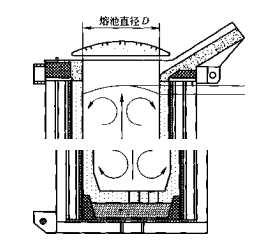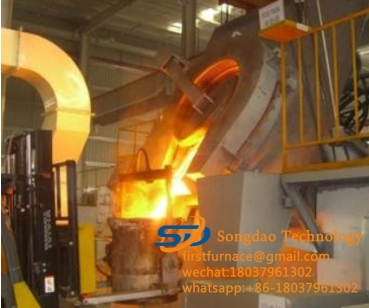### The top of the molten pool of the induction melting furnace forms a “hump” working principle

The top of the molten pool of the induction melting furnace forms a “hump” working principle

In the smelting process of the induction melting furnace, once the metal material is melted, the melt will form a regular movement under the action of electromagnetic force. This movement starts from the center of the molten pool and moves to both ends of the coil. Because the metal is constrained by the furnace bottom and the furnace wall, the final movement is always upward, forming a “hump” at the top of the molten pool. Some data use the ratio of the height of the hump to the diameter of the molten pool to express the stirring strength of the molten pool. The appearance of the “hump” is shown in Figure 2-9.Figure 2-9 Schematic diagram of the “hump” morphology of the melt in the induction melting furnace

However, in order to accurately express the shape of the “hump” of the induction melting furnace, and to reveal the flow and deformation behavior of liquid metal under the action of electromagnetic field, it is necessary to solve the Maxwell equations (coupled with Ohm’s law) to obtain the electromagnetic force. The electromagnetic force of is substituted into Navier-Stokes equation and continuity equation as volume force to obtain the flow velocity and free surface shape. At the same time, when the free surface shape of the melt changes, it will inevitably affect the distribution of the electromagnetic field in the melt, and then affect the electromagnetic force acting in the melt, which will change the free surface shape and velocity distribution of the melt. , It can be seen that the flow field and the electromagnetic field are highly coupled.

In order to obtain the morphology of the melt “hump” in the equilibrium state and simplify the calculation process, the following basic assumptions can be made for the induction melting furnace:

(1) Due to the skin effect, the current penetration depth 3 is much smaller than the size of the increase and the metal melt. Therefore, the electromagnetic force acting in the melt can be regarded as a surface force and can be expressed by a magnetic stress tensor (magnetic stress tensor);

(2) The morphological change of the melt “hump” does not affect the distribution of magnetic lines of force in the melt;

(3) If it is a split copper snail, since the electromagnetic field can only enter the melt through the gap between the split lobes, the end effect of the electromagnetic field is very small. Therefore, the electromagnetic induction in the split copper increase The intensity is calculated according to the electromagnetic induction intensity inside the infinite solenoid. When the system reaches equilibrium, the surface tension on the hump, the static pressure of the melt and the instantaneous average equivalent electromagnetic surface force reach equilibrium.